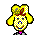"PIC'n Up The Pace"
PIC Microcontroller Applications GuideWanda says the initial reviews have been very good and people have indicated "PIC'n Up The Pace" is the logical next step after "Easy PIC'n."INTRODUCTION

PARTS IS PARTS

PROGRAMMING STYLE

Easy to understand code vs. short code vs. execution time

CIRCUIT FOR EXPERIMENTS

USING OP-AMPS

SERIAL COMMUNICATION

Overview
SHIFT REGISTERS
Serial in, parallel out 74HC164
Parallel in, serial out 74HC165 Use as input ports
Serial in, parallel out 74HC595 Use as output ports

SERIAL EEPROMS

93C46 3-wire

PIC-TO-PIC SERIAL COMMUNICATION

Bit banging, do it yourself simple serial interface

LCD INTERFACE

1x16 Alphanumeric LCD
Test circuit
Parallel, 8-bit mode
Initial test code
Display hex byte
Parallel, 4 bit mode
Initial test code
Serial Interface
D/A CONVERSION
Do it yourself using a resistor network
Do it yourself PIC16/17 Pin and PWM
PWM basics
Low pass filter
Analog output - software PWM - Increase/decrease buttons
PWM using TMR0
8-bit serial MAX 522 3-wire
Output a voltage level
Output a voltage ramp via increment counter
Output sine wave via table

SENSORS - ANALOG VOLTAGE OUTPUT (EXAMPLES)

Potentiometer for simulation
Temperature measurement - LM335
Offset and scale
+/- supplies, 3 op-amps, Vref
Single supply, 1 op-amp

A/D CONVERSION

Fundamentals
PIC16 Pin and RC time constant
Measuring resistance
Measuring voltage
Pot input
Temperature measurement - LM335, offset and scale
16C71 on-board A/D
MATH ROUTINES
Instructions required
Arithmetic
Subtraction
Multiplication
Multiply 1-byte binary number by 10 decimal subroutine
Double precision
Subtraction
Multiplication
Multiply 2-byte binary number by 10 decimal subroutine
8-Bit x 8-Bit multiply, 2 byte result
DECIMAL INTERFACE
Decimal to binary conversion
3-digit decimal to 8-Bit binary
Binary to decimal conversion
8-bit binary to 3-digit BCD
Displaying 8-bit binary result as a 3-digit decimal number on LCD -leading zero suppression
16-bit binary to 5-digit BCD
DIGITAL THERMOMETER - BRING IT ALL TOGETHER
Building blocks
Calculating temperature
Decimal round-off routine
Displaying temperture on LCD - leading zero suppression and rounding off
PIC16F84 EEPROM DATA MEMORY

PROGRAM MEMORY PAGING

LOCATING TABLES IN PROGRAM MEMORY

[an error occurred while processing this directive]